# Select first or last N rows in a Dataframe using head() and tail() method in Python-Pandas

Let’s discuss how to select top or bottom N number of rows from a Dataframe using head() & tail() methods.

1) Select first N Rows from a Dataframe using `head()` method of Pandas DataFrame :

Pandas `head()` method is used to return top n (5 by default) rows of a data frame or series

Parameters: (optional) n is integer value, number of rows to be returned.

Return: Dataframe with top n rows .

Let’s Create a dataframe

 `# import pandas library as pd ` `import` `pandas as pd ` ` `  `# List of Tuples ` `students ``=` `[(``'Ankit'``, ``22``, ``'Up'``, ``'Geu'``), ` `           ``(``'Ankita'``, ``31``, ``'Delhi'``, ``'Gehu'``), ` `           ``(``'Rahul'``, ``16``, ``'Tokyo'``, ``'Abes'``), ` `           ``(``'Simran'``, ``41``, ``'Delhi'``, ``'Gehu'``), ` `           ``(``'Shaurya'``, ``33``, ``'Delhi'``, ``'Geu'``), ` `           ``(``'Harshita'``, ``35``, ``'Mumbai'``, ``'Bhu'` `), ` `           ``(``'Swapnil'``, ``35``, ``'Mp'``, ``'Geu'``), ` `           ``(``'Priya'``, ``35``, ``'Uk'``, ``'Geu'``), ` `           ``(``'Jeet'``, ``35``, ``'Guj'``, ``'Gehu'``), ` `           ``(``'Ananya'``, ``35``, ``'Up'``, ``'Bhu'``) ` `            ``] ` ` `  `# Create a DataFrame object from ` `# list of tuples along with columns ` `# and indices. ` `details ``=` `pd.DataFrame(students, columns ``=``[``'Name'``, ``'Age'``, ` `                                           ``'Place'``, ``'College'``], ` `                        ``index ``=``[``'a'``, ``'b'``, ``'c'``, ``'d'``, ``'e'``, ` `                                ``'f'``, ``'g'``, ``'i'``, ``'j'``, ``'k'``]) ` ` `  `details `

Output: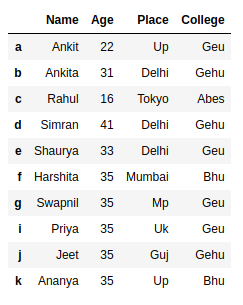Example 1:

 `# Show first 5 rows of the details dataframe ` `# from top ` `details.head() `

Output: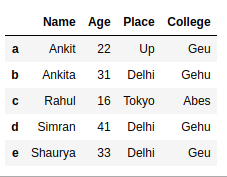Example 2:

 `# display top 3 rows of the dataframe ` `details.head(``3``) `

Output: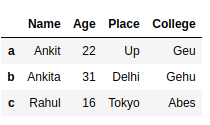Example 3:

 `# display top 2 rows of the specific columns ` `details[[``'Name'``, ``'Age'``]].head(``2``) `

Output: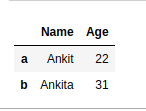2) Select last N Rows from a Dataframe using tail() method of Pandas DataFrame :

Pandas `tail()` method is used to return bottom n (5 by default) rows of a data frame or series.

Syntax: Dataframe.tail(n)

Parameters: (optional) n is integer value, number of rows to be returned.

Return: Dataframe with bottom n rows .

Example 1:

 `# Show bottom 5 rows of the dataframe ` `details.tail() `

Output: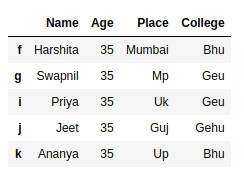Example 2:

 `# Show bottom 3 rows of the dataframe ` `details.tail(``3``) `

Output: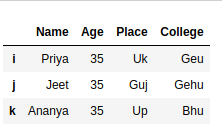Example 3:

 `# Show bottom 2 rows of the specific ` `# columns from dataframe ` `details[[``'Name'``, ``'Age'``]].tail(``2``) `

Output: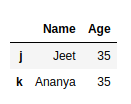Attention geek! Strengthen your foundations with the Python Programming Foundation Course and learn the basics.

To begin with, your interview preparations Enhance your Data Structures concepts with the Python DS Course.

My Personal Notes arrow_drop_upCheck out this Author's contributed articles.

If you like GeeksforGeeks and would like to contribute, you can also write an article using contribute.geeksforgeeks.org or mail your article to contribute@geeksforgeeks.org. See your article appearing on the GeeksforGeeks main page and help other Geeks.

Please Improve this article if you find anything incorrect by clicking on the "Improve Article" button below.

Article Tags :

Be the First to upvote.

Please write to us at contribute@geeksforgeeks.org to report any issue with the above content.